### Python Libraries: Numpy and Scipy

Numpy is a Python library for numerical computation using numbers. To use Numpy, it must first be imported as follows:

import numpy as np

## Numpy Matrices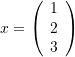To express the above matrix in Numpy, use array():

import numpy as np
x = np.array([1,2,3])
print(x)

Note that numpy arrays are similar but not exactly the same as Python lists

To express larger matrices like the matrix M below, each row in the matrix would be defined between two square brackets, individual rows are separated by commas and enclosed by a set of square brackets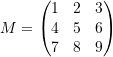import numpy as np
x = np.array([[1,2,3],[4,5,6],[7,8,9]])
print(x)

### Matrix Math Operations

Let x and y be two unique numpy vectors, let M be a numpy matrix, and let n be a constant

• Dot Product: np.dot(x,y)
• Cross Product: np.cross(x,y)
• Multiplication of a Matrix With a Vector: np.matmul(M,x)
• Multiplication of Matrices: np.matmul(M,M)
• Scalar Multiplication: n*x
• Addition of Matrices: x+y
• Determinant: np.linalg.det(x)
• Inverse: np.linalg.inv(x)
• Transpose: x.T
• Exponential: x**n
import numpy as np

M = np.array([[1,2,3],[4,5,6],[7,8,9]])
x = np.array([1,2,3])
y = np.array([4,5,6])
print("Dot Product:",np.dot(x,y))       # 32
print("Cross Product:",np.cross(x,y))   # [-3  6 -3]
print("Multiplication of a Matrix With a Vector:",np.matmul(M,x))
# [14 32 50]
print("Multiplication of Matrices:\n",np.matmul(M,M))
# [[30 36 42][66 81 96][102 126 150]]
print("Scalar Multiplication:",2*x)     # [2 4 6]
print("Addition of Matrices:",x+y)      # [5 7 9]

M = np.array([[1, 2],[3, 4]])
print("Determinant:",np.linalg.det(M))  # -2
print("Inverse:\n",np.linalg.inv(M))    # [[-2 1][1.5 -0.5]]
print("Transpose:\n",M.T)               # [[1 3][2 4]]
print("Exponential:\n",M**2)            # [[1 4][9 16]]

### Matrix Access Operations

Let M be a matrix and let r be the row number and c be the column number

• Get Row r: M[r,:]
• Get Column c: M[:,c]
• Element at (r,c): M[r,c]
import numpy as np

M = np.array([[1,2,3],[4,5,6],[7,8,9]])
print("M:",M)                     # [[1 2 3][4 5 6][7 8 9]]
print("Row 0:",M[0,:])            # [1 2 3]
print("Col 1:",M[:,1])            # [2 5 8]
print("Element at (1,2):",M[1,2]) # 6

Notice that elements in numpy matrices are accessed as M[r,c], whereas list matrices are accessed as M[r][c]

### Matrix Manipulation Operations

Let M be a matrix, let r be the row number, let c be the column number, and let n be a constant

• Insert to Row r: M = np.insert(M, r, np.array([[...]]), axis=0)
• Insert to Column c: M = np.insert(M, c, np.array([...]), axis=1)
• Delete Row r: M = np.delete(M, r, axis=0)
• Delete Column c: M = np.delete(M, c, axis=1)
import numpy as np

M = np.array([[1,2,3],[4,5,6],[7,8,9]])
display("M:",M)
M = np.insert(M, 0, np.array([[9,9,9]]), axis=0)
display("Insert to Row 0:",M)
M = np.insert(M, 1, np.array([0,0,0,0]), axis=1)
display("Insert to Col 1:",M)
M = np.delete(M, 0, axis=0)
display("Delete Row 0:",M)
M = np.delete(M, 1, axis=1)
display("Delete Col 1:",M)

## Numpy Built In Functions

Let x,a,b,n be numbers

• Pi: np.pi
• Infinity: np.inf
• Sine: np.sin(x)
• Cosine: np.cos(x)
• Log: np.log(x)
• Exponential: np.exp(x)
• Factorial: np.math.factorial(x)
• Square Root: np.sqrt(x)
• List of n Zeros: np.zeros(n)
• Matrix of Zeros: np.zeros([a,b])
• Sum: np.sum([a,b])
• Linspace: np.linspace(a,b,n)
• Array of n evenly spaced numbers between a and b
• Arange: np.arange(a,b,n)
• Array of evenly spaced numbers between a and b with a step size n
import numpy as np

print("Pi:",np.pi)
print("Infinity:",np.inf)
print("Sine:",np.sin(5))
print("Cosine:",np.cos(5))
print("Log:",np.log(5))
print("Exponential:",np.exp(5))
print("Factorial:",np.math.factorial(5))
print("Square Root:",np.sqrt(5))
print("Zeros List:",np.zeros(3))
print("Zeros Matrix:",np.zeros([3,4]))
print("Sum:",np.sum([1,2,3]))
print("Linspace:",np.linspace(0,10,50))
print("Arange:",np.arange(0,10,0.2))

### Scipy Solving Equations

Scipy is a Python library that has functions for solving equations numerically

All solvers in Scipy require the system of equations to be the return value of a user-defined function and all unknown variables must have an initial guess. Therefore, these solvers will only return the set of solutions closest to the initial guesses

Scipy’s root and fsolve solves systems of linear and nonlinear equations

#### root

from scipy.optimize import root
def f(x):
vars = x
return [equations]
sol = root(f,[initial guesses])

#### fsolve

from scipy.optimize import fsolve
def f(x):
vars = x
return [equations]
sol = fsolve(f,[initial guesses])

Consider the following linear equation, and find the values of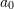,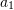, and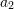that would satisfy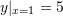,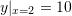, and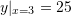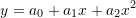Therefore, solve the following system of equations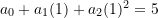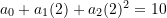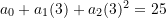def f(x):
a0, a1, a2 = x
x = 1; y1 = a0 + a1*x + a2*x**2 - 5
x = 2; y2 = a0 + a1*x + a2*x**2 - 10
x = 3; y3 = a0 + a1*x + a2*x**2 - 25
return [y1, y2, y3]

from scipy.optimize import root
sol = root(f,[0,0,0])
print("root:",sol.x)  # [10. -10. 5.]

from scipy.optimize import fsolve
sol = fsolve(f,[0,0,0])
print("fsolve:",sol)  # [10. -10. 5.]

Consider the following non-linear equation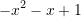def f(x):
x = x
return [-x**2 - x + 1]

from scipy.optimize import root
sol1 = root(f,[-1])
sol2 = root(f,)
print("root 1:",sol1.x)  # -1.61803...
print("root 2:",sol2.x)  # 0.61803...

from scipy.optimize import fsolve
sol1 = fsolve(f,[-1])
sol2 = fsolve(f,)
print("fsolve 1:",sol1)  # -1.61803...
print("fsolve 2:",sol2)  # 0.61803...